# How to quickly find the maximum and minimum values in Excel [Tip]

If you need to find the maximum and minimum values from a range of Excel spreadsheet cells, the MAX and MIN functions will come in handy. The MAX function returns the highest value from a range of cells. You can add the MIN function to find the lowest value from a cell range.

As an example enter 5, 6 and 7 into an Excel spreadsheet column. Then select a cell to add the function to, and click the Insert Function (fx) button. That will open the Insert Function window in the shot below.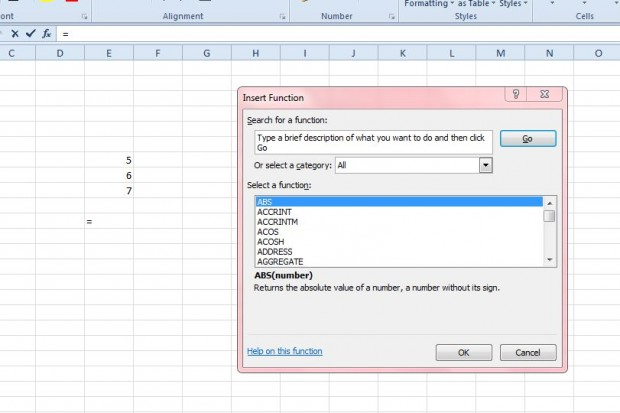Then select MAX from that window. That will open the window shown in the shot directly below. There press the button beside the Number1 text box, and select the three cells that include the numbers in them.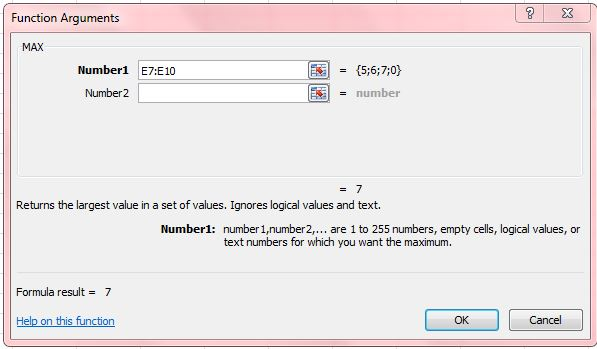Press OK to close the window. The spreadsheet will then show you the maximum value from the range of cells selected as below.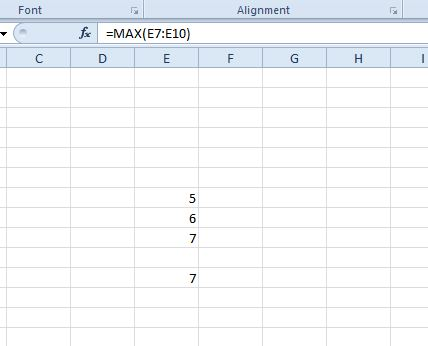You can find the minimum value from a range of cells much the same. Select MIN from the Insert Function window. That will open the window in the shot below from which you can select the range of cells to find the minimum value.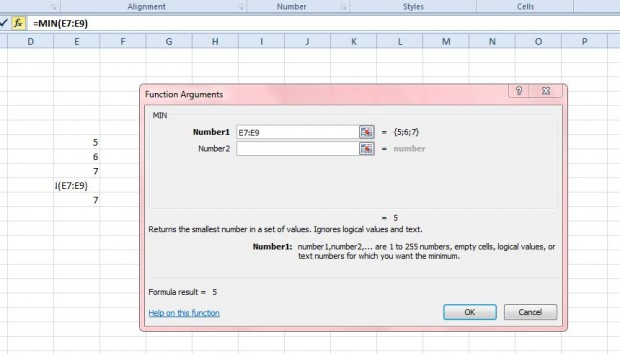In addition, you can also add MAXA and MINA functions to an Excel spreadsheet. Those are functions that consider text values entered into cells such as erue and false. True equals one and false equals zero.

Enter true and false into a couple of spreadsheet cells. Then click a cell to add the MAXA or MINA function to, and select one from the Insert Function window as before. Next, select the range of cells that include true and false; and click OK to close the window. If you added the MAXA function to the spreadsheet, it will show one as the maximum value as below.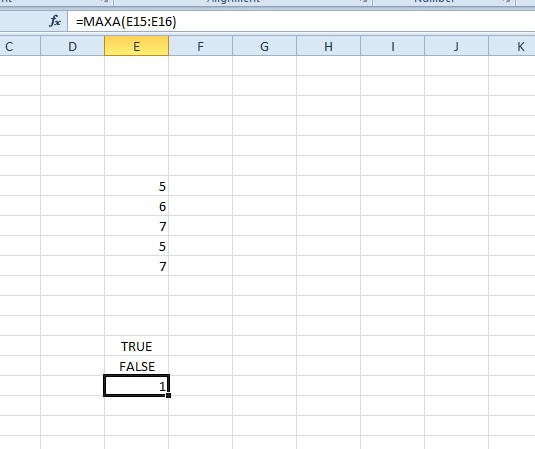These functions will probably not be needed to find maximum and minimum values from just three cells as in the examples above. However, what if you have a spreadsheet with hundreds of alternative cell values in it? Then it might take a while to find the max and min values from a larger range of cells, and that’s when the functions above will certainly come in handy.